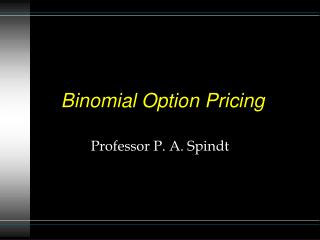# Binomial Option Pricing - PowerPoint PPT PresentationDownload PresentationBinomial Option Pricing

Binomial Option PricingDownload Presentation## Binomial Option Pricing

- - - - - - - - - - - - - - - - - - - - - - - - - - - E N D - - - - - - - - - - - - - - - - - - - - - - - - - - -
##### Presentation Transcript

1. Binomial Option Pricing Professor P. A. Spindt

2. A simple example • A stock is currently priced at \$40 per share. • In 1 month, the stock price may • go up by 25%, or • go down by 12.5%.

3. A simple example • Stock price dynamics: t = now t = now + 1 month up state \$40x(1+.25) = \$50 \$40 \$40x(1-.125) = \$35 down state

4. Call option • A call option on this stock has a strike price of \$45 t=0 t=1 Stock Price=\$50; Call Value=\$5 Stock Price=\$40; Call Value=\$c Stock Price=\$35; Call Value=\$0

5. A replicating portfolio • Consider a portfolio containing Dshares of the stock and \$B invested in risk-free bonds. • The present value (price) of this portfolio is DS + B = \$40 D + B

6. \$50 D + (1+r/12)B \$40 D + B \$35 D + (1+r/12)B Portfolio value t=0 t=1 up state down state

7. A replicating portfolio • This portfolio will replicate the option if we can find a D and a B such that Up state \$50 D + (1+r/12) B = \$5 and Down state \$35 D + (1+r/12) B = \$0 Portfolio payoff Option payoff =

8. The replicating portfolio • Solution: • D = 1/3 • B = -35/(3(1+r/12)). • Eg, if r = 5%, then the portfolio contains • 1/3 share of stock (current value \$40/3 = \$13.33) • partially financed by borrowing \$35/(3x1.00417) = \$11.62

9. The replicating portfolio • Payoffs at maturity

10. The replicating portfolio • Since the the replicating portfolio has the same payoff in all states as the call, the two must also have the same price. • The present value (price) of the replicating portfolio is \$13.33 - \$11.62 = \$1.71. • Therefore, c = \$1.71

11. A general (1-period) formula

12. An observation about D • As the time interval shrinks toward zero, delta becomes the derivative.

13. Put option • What about a put option with a strike price of \$45 t=0 t=1 Stock Price=\$50; Put Value=\$0 Stock Price=\$40; Put Value=\$p Stock Price=\$35; Put Value=\$10

14. \$50 D + (1+r/12)B \$40 D + B \$35 D + (1+r/12)B A replicating portfolio t=0 t=1 up state down state

15. A replicating portfolio • This portfolio will replicate the put if we can find a D and a B such that Up state \$50 D + (1+r/12) B = \$0 and Down state \$35 D + (1+r/12) B = \$10 Portfolio payoff Option payoff =

16. The replicating portfolio • Solution: • D = -2/3 • B = 100/(3(1+r/12)). • Eg, if r = 5%, then the portfolio contains • short 2/3 share of stock (current value \$40x2/3 = \$26.66) • lending \$100/(3x1.00417) = \$33.19.

17. Two Periods Suppose two price changes are possible during the life of the option At each change point, the stock may go up by Ru% or down by Rd%

18. Two-Period Stock Price Dynamics • For example, suppose that in each of two periods, a stocks price may rise by 3.25% or fall by 2.5% • The stock is currently trading at \$47 • At the end of two periods it may be worth as much as \$50.10 or as little as \$44.68

19. Two-Period Stock Price Dynamics \$50.10 \$48.53 \$47 \$47.31 \$45.83 \$44.68

20. Terminal Call Values At expiration, a call with a strike price of \$45 will be worth: Cuu =\$5.10 \$Cu \$C0 Cud =\$2.31 \$Cd Cdd =\$0

21. Two Periods The two-period Binomial model formula for a European call is

22. Example TelMex Jul 45 143 CB 23/16 -5/16 472,703

23. Estimating Ru and Rd According to Rendleman and Barter you can estimate Ru and Rd from the mean and standard deviation of a stock’s returns

24. Estimating Ru and Rd In these formulas, t is the option’s time to expiration (expressed in years) and n is the number of intervals t is carved into

25. For Example • Consider a call option with 4 months to run (t = .333 yrs) and • n = 2 (the 2-period version of the binomial model)

26. For Example • If the stock’s expected annual return is 14% and its volatility is 23%, then

27. For Example • The price of a call with an exercise price of \$105 on a stock priced at \$108.25

28. Anders Consulting • Focusing on the Nov and Jan options, how do Black-Scholes prices compare with the market prices listed in case Exhibit 2? • Hints: • The risk-free rate was 7.6% and the expected return on stocks was 14%. • Historical Estimates: sIBM = .24 & sPepsico = .38